# Motives, theory of

(diff) ← Older revision | Latest revision (diff) | Newer revision → (diff)

A generalization of the various cohomology theories of algebraic varieties. The theory of motives systematically generalizes the idea of using the Jacobian of an algebraic curveas a replacement for the cohomology groupin the classical theory of correspondences, and the use of this theory in the study of the zeta-function of a curveover a finite field. The theory of motives is universal in the sense that every geometric cohomology theory, of the type of the classical singular cohomology for algebraic varieties overwith constant coefficients, every-adic cohomology theory for various prime numbersdifferent from the characteristic of the ground field, every crystalline cohomology theory, etc. (see Weil cohomology) are functors on the category of motives.

Let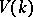be the category of smooth projective varieties over a fieldand let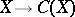be a contravariant functor of global intersection theory frominto the category of commutative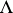-algebras, whereis a fixed ring. For example,is the Chow ring of classes of algebraic cycles (cf. Algebraic cycle) onmodulo a suitable (rational, algebraic, numerical, etc.) equivalence relation, oris the Grothendieck ring, or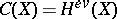is the ring of cohomology classes of even dimension, etc. The categoryand the functorenable one to define a new category, the category of correspondences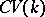, whose objects are varieties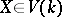, denoted by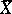, and whose morphisms are defined by the formulawith the usual composition law for correspondences (see ). Let the functortake values in the category of commutative graded-algebras. Thenwill be the-additive category of graded correspondences. Moreover,will have direct sums and tensor products.

The category whose objects are the varieties fromand whose morphisms are correspondences of degreeis denoted by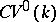. A natural functor fromintohas been defined, and the functorextends to a functorfromto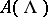. The category, like, is not Abelian. Its pseudo-Abelian completion, the category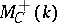, has been considered. It is obtained fromby the formal addition of the images of all projections. More precisely, the objects ofare pairs, whereand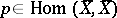,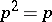, and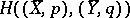is the set of correspondencessuch that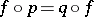modulo a correspondencewith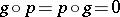. The categoryis imbedded inby means of the functor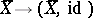. The natural functor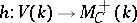is called the functor of motive cohomology spaces andis called the category of effective motives.

Let, whereis the class of any rational point on the projective line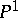, and let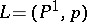. Then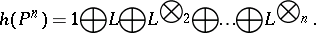Ifis the projectivization of a locally free sheafof rankon, then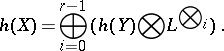Motives of a monodial transformation with a non-singular centre, motives of curves (see ), motives of Abelian manifolds (see ), and motives of Weil hypersurfaces have also been calculated.

The category of motives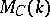is obtained fromby the formal addition of negative powers of the motives. By analogy with-adic cohomology,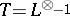is called the Tate motive. Tensor multiplication withis called twisting by the Tate motive. Twisting enables one to define the level of a motive as in an-adic cohomology theory. Any functor of the Weil cohomology factors through the functor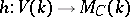. There is the conjecture thatdoes not, in some sense, depend on the intersection theory of, and that the functoris itself a (universal) theory for the Weil cohomology. This conjecture is closely related to the standard Grothendieck conjectures (see ) on algebraic cycles (at present, 1982, not proved).

How to Cite This Entry:
Motives, theory of. Encyclopedia of Mathematics. URL: http://encyclopediaofmath.org/index.php?title=Motives,_theory_of&oldid=16124
This article was adapted from an original article by V.A. Iskovskikh (originator), which appeared in Encyclopedia of Mathematics - ISBN 1402006098. See original article